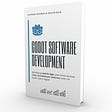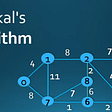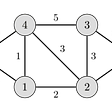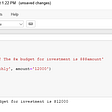# Find Odd Occurring Element Using XOR

This is just one of the cool things that you can do with XOR.

# Problem Statement

Given an array, find number that occurs odd number of times.

`Input: [1,2,2,3,3,1,5,1,5,5,5]Output: 1`

# Understanding XOR (eXclusive OR)

The logic is simple. If the bits are the same, the result is `0`. If the bits are different, the result is `1`.

You can use this calculator to try out some numbers yourself.

Let’s look at a few examples. Take the number 10 and 9.

The XOR of 10 and 9 is 3 (binary representation of `011`).

What if we take the same number and do an XOR?

We will get `0` as a result!

Now what if we do an XOR between any number(i.e. 10) and a `0`?

We get back the same number that we put in.

So, it means if we do an XOR on two same numbers(i.e. that number occurs even times), we will get a `0` . If we do an XOR between any number and `0`, it would not change that number in any way.

To conclude:

`// Remember b ^ b cancel each others outa = a ^ (b ^ b)// b ^ 0 will not change bb = b ^ 0`

# Solution: Find odd occurring element using XOR

Assuming there is only one number that occurs odd number of times, it means all other numbers occur even number of times. If we do an XOR on the number that occurs even number of times, they will cancel out each others!

The number that will be left would be the one that occurs odd number of times.

# Space and time complexity

Space complexity is `O(1)` as we use constant space.

Time complexity is `O(n)` as we loop through the entire input array once.

--

--

--

## More from Felix Lee

Software engineer in Singapore. Write random tech stuff. Still figuring out this “life” thing in life.

Love podcasts or audiobooks? Learn on the go with our new app.

## How I doubled my productivity as a Software developer## Can you hack your time in order to learn making games?## Multiprocessing in Python: an example## Is Trigger?## ARK Messenger Tutorial — Part One## Debugging Python code## Felix Lee

Software engineer in Singapore. Write random tech stuff. Still figuring out this “life” thing in life.

## Kruskal’s Algorithm## Cheat Sheet for Array methods in JS## Concept Of Template and Do while loop in Python